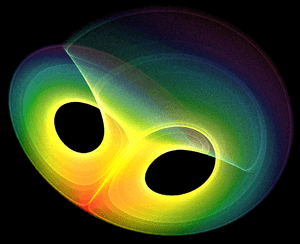# Mathematical analysis facts for kids

Kids Encyclopedia FactsA strange attractor arising from a differential equation. Differential equations are an important area of mathematical analysis with many applications to science and engineering.

Mathematical analysis is a part of mathematics. It is often shortened to analysis. It looks at functions, sequences and series. These have useful properties and characteristics that can be used in engineering. The mathematical analysis is about continuous functions, differential calculus and integration.

Gottfried Wilhelm Leibniz and Isaac Newton developed most of the basis of mathematical analysis.

## Other pages

Some topics in analysis are:

Some useful ideas in analysis are:Mathematical analysis Facts for Kids. Kiddle Encyclopedia.